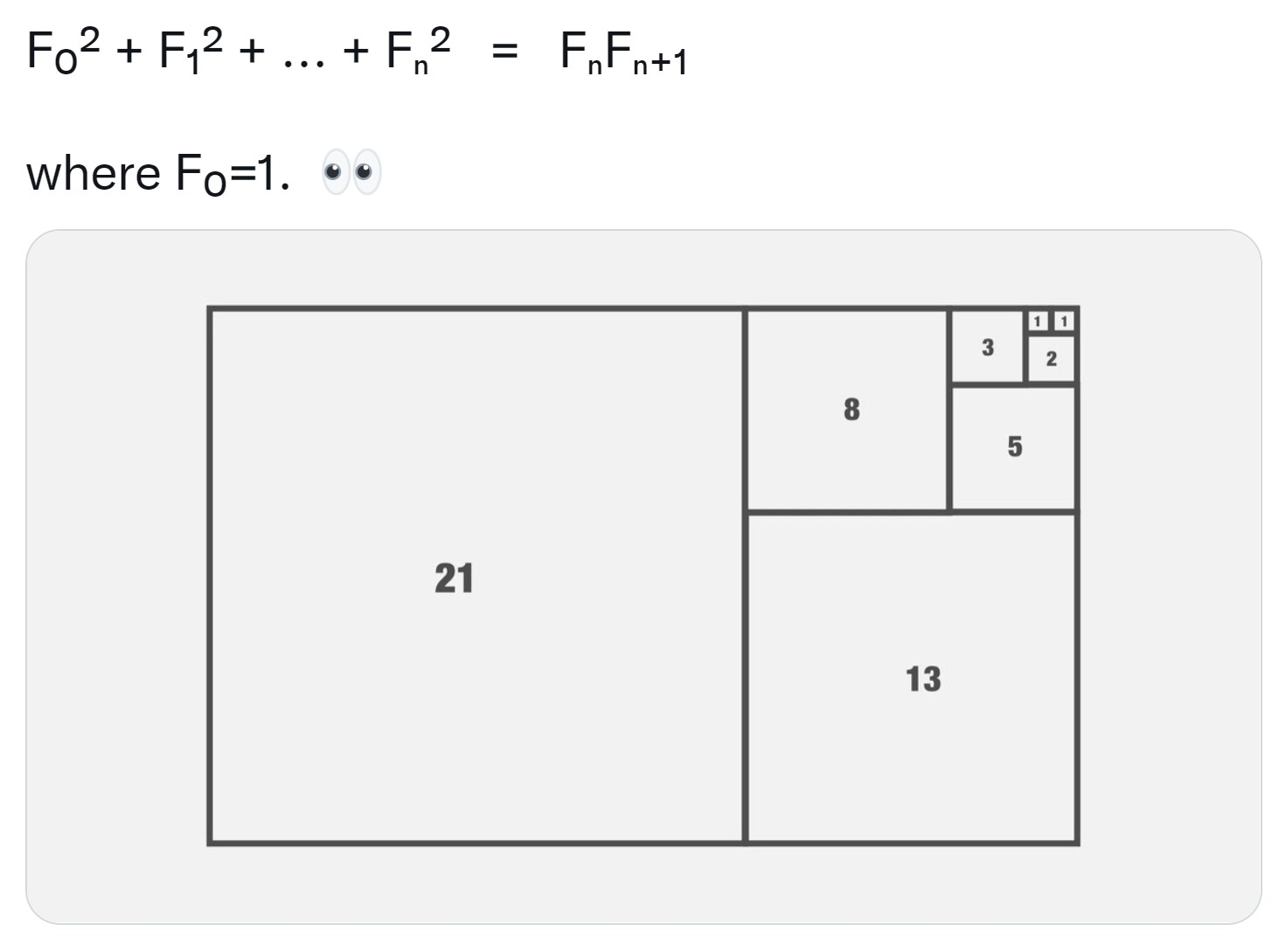# A visual proof that the sum of the squares of the first Fibonacci numbers up to Fₙ is the product of the nth and (n+1)th Fibonacci numbers[From here.]